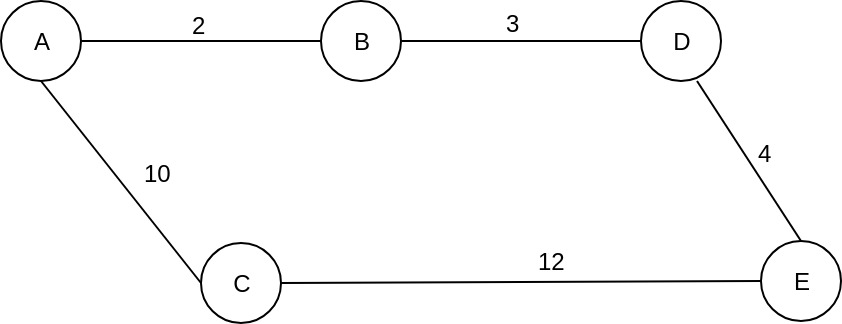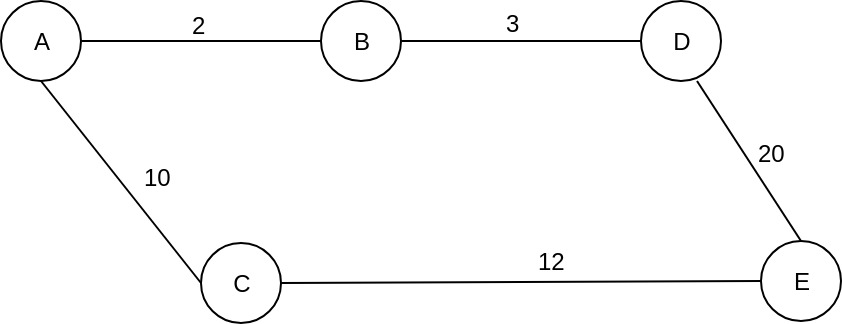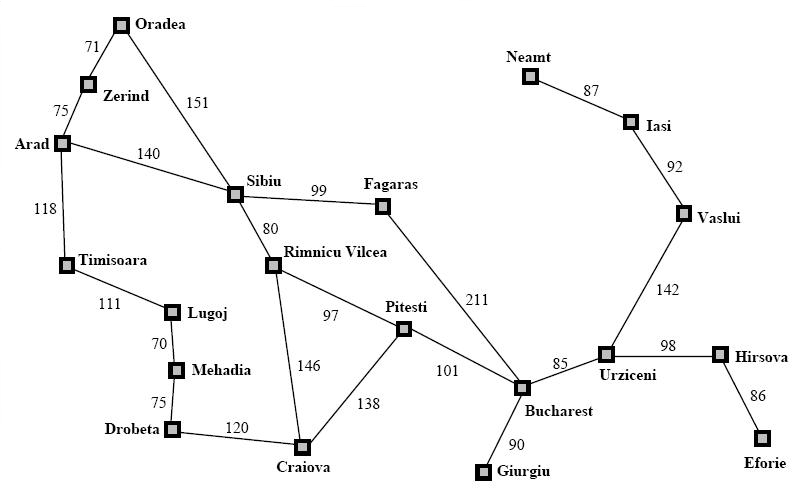## 在正加权图中寻找最短路径``````Queue: {
B, cost_so_far: 2
C, cost_so_far: 10
}``````

``````Queue {
C, cost_so_far: 10,
D, cost_so_far: 5
}``````

``````Queue {
E, cost_so_far: 14
C, cost_so far: 10
}````````````Queue {
E, cost_so_far: 25
C, cost_so far: 10
}``````

### 迪伊克斯特拉实施建议

``````static class Node{
public final String name;
public List<Edge> adjacency = new ArrayList<>();
public Node parent;
public double g_cost = Integer.MAX_VALUE;

public Node(String name){
this.name = name;
}

public void addNeighbour(Node neighbour, int cost) {
Edge edge = new Edge(neighbour, cost);
adjacency.add(edge);
}

public String toString(){
return name;
}
}``````

``````static class Edge{
public final double cost;
public final Node target;

public Edge(Node target, double cost){
this.target = target;
this.cost = cost;
}
}``````

``````public static void dijkstraSearch(Node source, Node destination) {
source.g_cost = 0;

PriorityQueue<Node> frontier = new PriorityQueue<>(new Comparator<Node>() {
@Override
public int compare(Node o1, Node o2) {
return Double.compare(o1.g_cost, o2.g_cost);
}
});

frontier.add(source);

int numberOfSteps = 0;
while (!frontier.isEmpty()) {
numberOfSteps++;
// get the node with minimum g_cost
Node current = frontier.poll();

System.out.println("Current node: " + current.name);

// we have found the destination
if (current.name.equals(destination.name)) {
break;
}

// check all the neighbours
for (Edge edge: current.adjacency) {

Node neigh = edge.target;
double cost = edge.cost;

double newCost = current.g_cost + cost;

if (newCost < neigh.g_cost) {
neigh.parent = current;
neigh.g_cost = newCost;

// reset frontier order
if (frontier.contains(neigh)) {
frontier.remove(neigh);
}

frontier.add(neigh);
}
}
}

System.out.println("Number of steps: " + numberOfSteps);
}````````````Total Cost: 418.0
Path: [Arad, Sibiu, Rimnicu Vilcea, Pitesti, Bucharest]``````

``````Current node: Arad
Current node: Zerind
Current node: Timisoara
Current node: Sibiu
Current node: Oradea
Current node: Rimnicu Vilcea
Current node: Lugoj
Current node: Fagaras
Current node: Mehadia
Current node: Pitesti
Current node: Craiova
Current node: Drobeta
Current node: Bucharest
Number of steps: 13``````

Comments are closed.